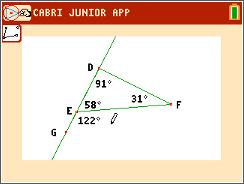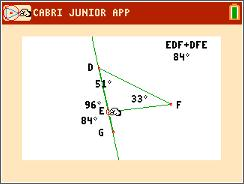••• ##### Device
• TI-84 Plus
• TI-84 Plus Silver Edition
•TI-84 Plus C Silver Edition
•TI-84 Plus CE
• ##### Software

TI Connect™ CE

• ##### Report an Issue

Geometry: Exterior & Remote Interior Angles
by Texas Instruments#### Overview

Students investigate an exterior angle and its two remote interior angles.

#### Key Steps

•Students are given a triangle with the interior angle measures. First they will construct and find the measure of an exterior angle of a triangle. When they drag a vertex of the triangle, students will record the measurements of the exterior angle and the two remote interior angles. They will discover the corollary to the Triangle Exterior Angle Theorem.

•Then students continue the exploration by using the Calculate tool to find the sum of the two remote interior angles. Comparing the sum to the measure of the exterior angle, students will discover the Triangle Exterior Angle Theorem.